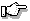# clock()

return the number of clock ticks used by the program

## Synopsis:

```#include <time.h>
clock_t clock(void);```

## Description:

The clock() function returns the number of clock ticks of processor time used by the program since the program started executing. This can be converted to seconds by dividing by the value of the macro CLOCKS_PER_SEC.The clock() function simply returns the difference between the current time and the time the program started, so it gives incorrect results if the system time changes somewhere between. The times() function provides more useful information.

## Returns:

The number of clock ticks that have occurred since the program started executing.

## Examples:

```#include <stdio.h>
#include <math.h>
#include <time.h>

void compute( void )
{
int i, j;
double x;

x = 0.0;
for( i = 1; i <= 100; i++ )
for( j = 1; j <= 100; j++ )
x += sqrt( (double) i * j );
printf( "%16.7f\n", x );
}

void main()
{
clock_t start_time, end_time;

start_time = clock();
compute();
end_time = clock();
printf( "Execution time was %lu seconds\n",
(end_time - start_time) / CLOCKS_PER_SEC );
}```

## Classification:

ANSI

Safety:
Interrupt handler No
Signal handler Yes